# Let AI play Connect4:Implementing using DQN neural network with myCobot 280 M5stack

• ## Introduction

Today, we shall acquaint ourselves with the subject of artificial intelligence in chess, whereby we shall employ a mechanical arm as an opponent to engage in a game of chess with you. The study of artificial intelligence in chess can be traced back to the 1950s, when computer scientists began to explore the writing of programs that would enable computers to play chess. The most renowned example of this was Deep Blue, developed by IBM, which in 1997 defeated the reigning world chess champion, Gary Kasparov, with a score of 3.5-2.5.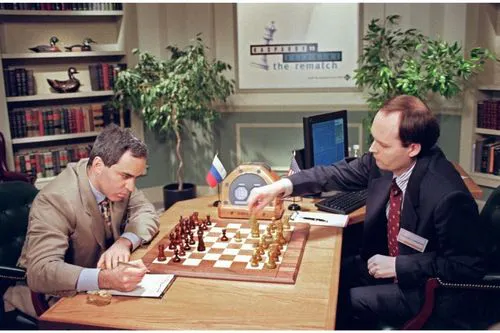Artificial intelligence in chess is akin to granting a computer a mode of deliberation that enables it to achieve victory in a match. There are many such modes, most of which stem from superior algorithms. At its core, Deep Blue's algorithm was based on brute-force search: generating every possible move, conducting searches as deeply as possible, constantly evaluating the game state, and attempting to identify the optimal move.
Now, let me show you how to realize the robot arm to play chess intelligently.

## Connect 4

The game I shall introduce today is known as Connect4, a strategic board game commonly referred to as "Four in a Row". The objective of Connect4 is to achieve a horizontal, vertical, or diagonal sequence of four game pieces within a vertically-oriented grid comprising six rows and seven columns. Two players take turns inserting their respective pieces from the top of the grid, with the pieces falling to the lowest available position within the selected column. Players may select any column in which to place their pieces, provided the pieces are placed only underneath existing pieces.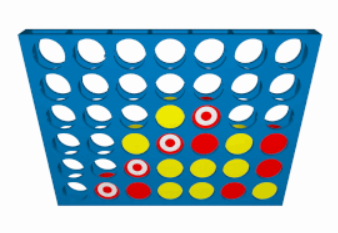As shown in the animation, this is Connect4.

## myCobot 280

The robotic arm selected for the task is the myCobot 280 M5Stack, a powerful desktop six-axis robot arm that employs the M5Stack-Basic as its control core and supports multiple programming languages. The myCobot 280's six-axis structure grants it high flexibility and precision, enabling it to perform a variety of complex operations and movements. It supports multiple programming languages, including Python, C++, and Java, allowing developers to program and control the mechanical arm according to their specific needs. Its user-friendly interface and detailed user manual facilitate rapid familiarization, while its embedded design ensures a compact size, making it easy to carry and store.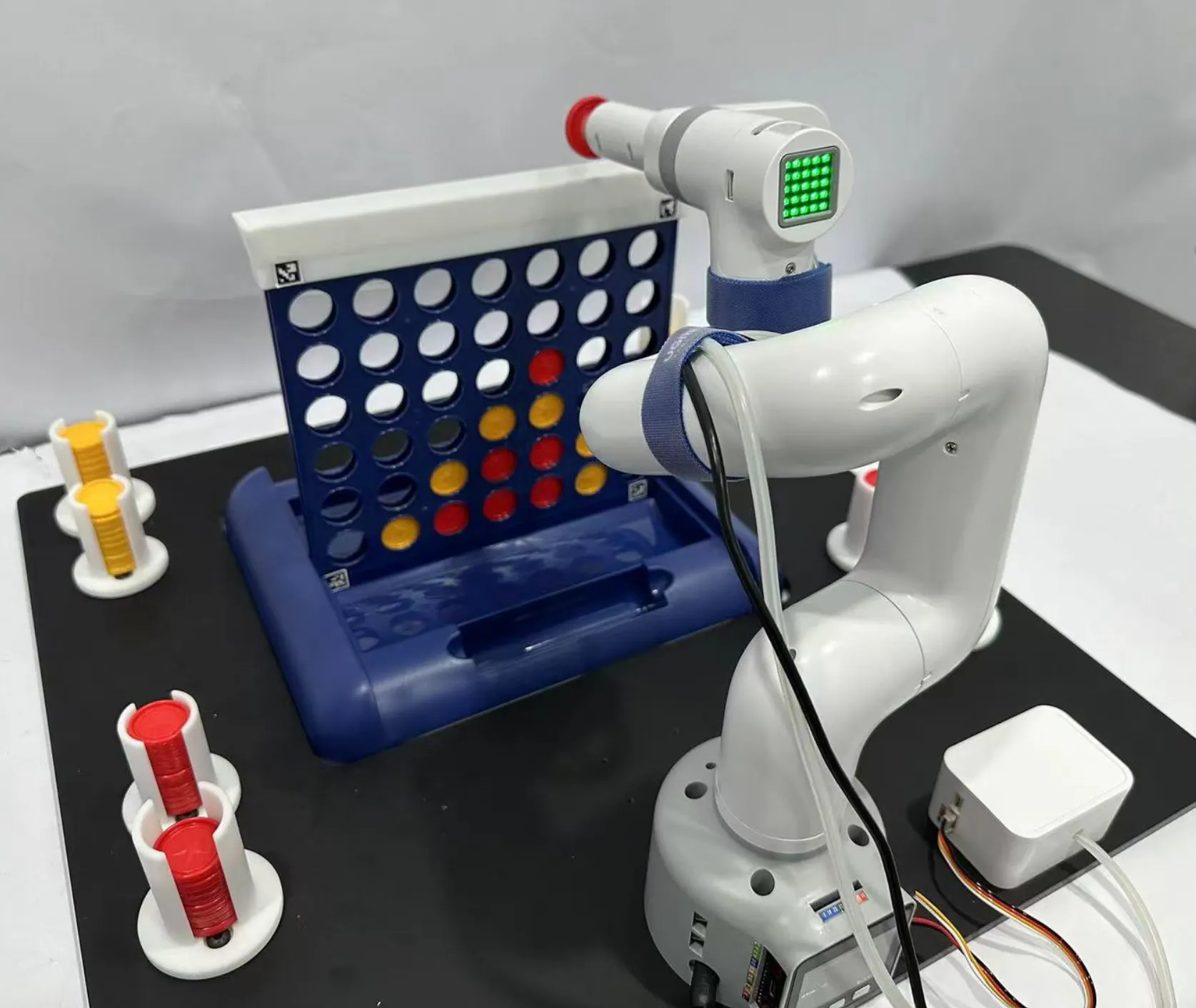This is the scene we built,we use myCobot280 to play connect4 with us.

## Algorithm for playing chess

Firstly, we must address the crucial matter of which algorithm to employ for playing chess. In other words, we must provide the mechanical arm with a cognitive brain capable of deep contemplation. Allow me to briefly present to you a few commonly used algorithms for playing chess:

### The Minimax Algorithm:

This is a classic game algorithm that is applicable for two-player games. It works by recursively simulating the moves of both the opponent and oneself, evaluating the score of every possible move, and selecting the action with the highest score. The Minimax Algorithm can find the best chess strategy by searching through the tree structure of the game board. This algorithm is a zero-sum game, meaning that one player chooses the option that maximizes their advantage from the available choices, while the other player selects the method that minimizes the advantage of their opponent. The total sum is zero at the beginning. Let me give a simple example of Tic-Tac-Toe to illustrate this.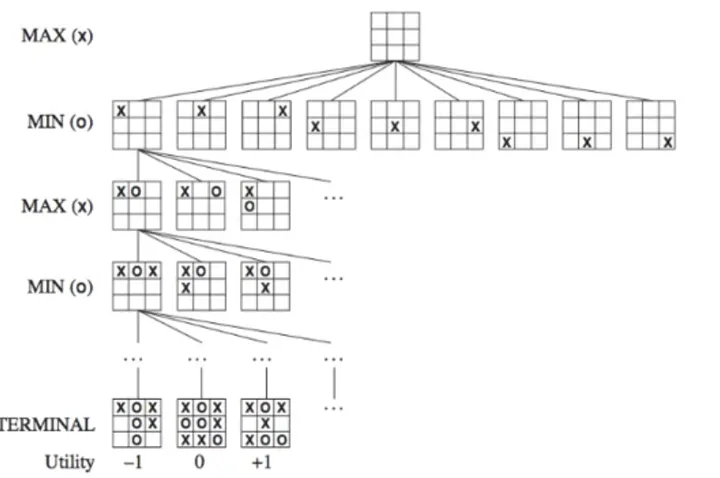Max represents us, while Min represents our opponent. At this point, we need to assign a score to every possible outcome, which is known as the Utility. This score is evaluated from our perspective (Max), for example, in the figure above, if I win, the score is +1, if I lose, it is -1, and if it is a tie, it is 0. Therefore, we want to maximize this score, while our opponent wants to minimize it. (In the game, this score is called the static value.) I must mention that Tic-Tac-Toe is a relatively simple game, so it is possible to list all possible outcomes. However, for most games, it is impossible to list all outcomes. According to the computational power of the computer, we may only be able to look forward to 7 or 8 steps, so the score is not as simple as -1, 1, 0. There will be specific algorithms to assign different scores based on the current outcome.

### Alpha-Beta Pruning Algorithm:

This is an optimization of the Minimax Algorithm. It reduces the number of branches to be searched by pruning, thus speeding up the search process. The Alpha-Beta Pruning Algorithm uses upper and lower bounds (Alpha and Beta values) to determine which branches can be discarded, reducing the depth of the search.
Neural Networks and Deep Learning:
The connect4 game algorithm that we designed also uses neural networks and deep learning for gameplay.

### Neural Networks:

Scientists have always hoped to simulate the human brain and create machines that can think. Why can humans think? Scientists have found that the reason lies in the neural network of the human body. Neural networks are mathematical models that simulate the structure and function of the human brain's nervous system. They process information and learn by simulating the connections and signal transmission between neurons. Neural networks are the foundation of all artificial intelligence.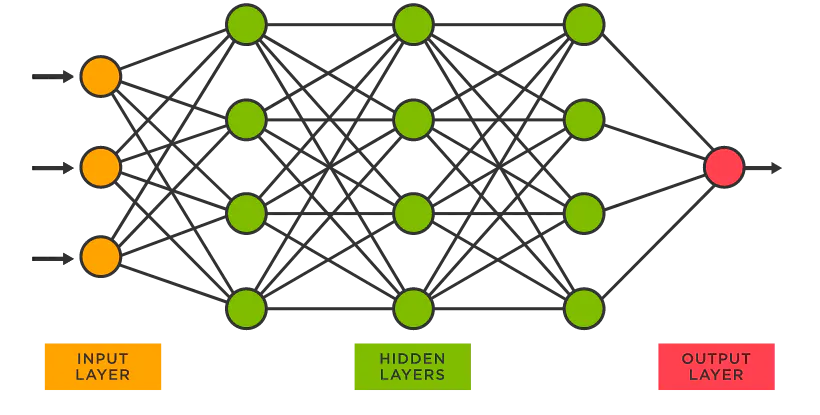The basic idea of the neural network algorithm is to pass input data to the input layer of the network, then obtain the output layer's results through a series of computations and transmissions in intermediate layers (hidden layers). The training process adjusts the connection weights to minimize the difference between the actual output and expected output, optimizing the performance of the network.
Deep Learning:
Deep learning is a branch of machine learning that focuses on using deep neural networks for learning and reasoning. Deep learning solves complex learning and decision-making problems by constructing deep neural networks, which have multiple intermediate layers (hidden layers). It can be said that deep learning is a learning method that uses neural networks as the core tool. Deep learning not only includes the structure and algorithm of neural networks but also involves training methods, optimization algorithms, and large-scale data processing.

## Project

The project is mainly divided into two parts, hardware and software: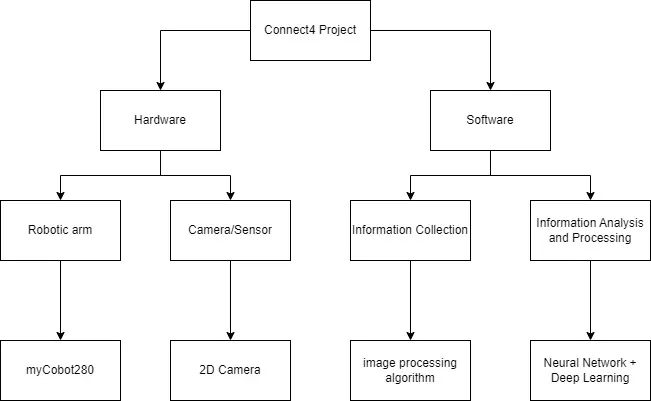The most crucial part of this project is information collection, analysis, and processing. As mentioned earlier, we also used relevant knowledge of neural algorithms and deep learning, and the specific neural network used is the DQN neural network.

### DQN Neural Network:

The DQN neural network was proposed by DeepMind and combines the ideas of deep learning and reinforcement learning. DQN uses a deep neural network to estimate the state-action value function (Q function), enabling optimal decision-making in complex environments. The core idea of DQN is to use a deep neural network as a function approximator to approximate the state-action value function. By taking the current state as input, the neural network outputs the corresponding Q value for each action, that is, predicting the long-term return of that action in the current state. Then, the optimal action is selected and executed based on the Q value.

### Environment Setup:

Firstly, we need to define the Connect4 game using a two-dimensional array to represent the game board and two types of game pieces, red (R) and yellow (Y). We also need to define the end condition of the game, which is when four game pieces of the same color are connected in a line, the game ends.

``````self.bgr_data_grid = [[None for j in range(6)] for i in range(7)]

#Used to display the state of the board
def debug_display_chess_console(self):
for y in range(6):
for x in range(7):
cell = self.stable_grid[x][y]
if cell == Board.P_RED:
print(Board.DISPLAY_R, end="")
elif cell == Board.P_YELLOW:
print(Board.DISPLAY_Y, end="")
else:
print(Board.DISPLAY_EMPTY, end="")
print()
print()
``````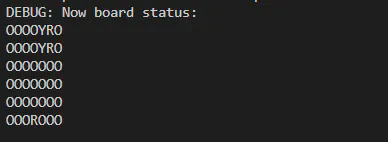Here is the code that defines whether the game is over:

``````def is_game_over(board):
# Check if there are four consecutive identical pieces in a row.
for row in board:
for col in range(len(row) - 3):
if row[col] != 0 and row[col] == row[col+1] == row[col+2] == row[col+3]:
return True

# Check if there are four consecutive identical pieces in a column.
for col in range(len(board)):
for row in range(len(board) - 3):
if board[row][col] != 0 and board[row][col] == board[row+1][col] == board[row+2][col] == board[row+3][col]:
return True

# Examine if there are four consecutive identical pieces in a diagonal line.
for row in range(len(board) - 3):
for col in range(len(board) - 3):
if board[row][col] != 0 and board[row][col] == board[row+1][col+1] == board[row+2][col+2] == board[row+3][col+3]:
return True

for row in range(len(board) - 3):
for col in range(3, len(board)):
if board[row][col] != 0 and board[row][col] == board[row+1][col-1] == board[row+2][col-2] == board[row+3][col-3]:
return True

# Verify if the game board is filled completely.
for row in board:
if 0 in row:
return False

return True
``````

Building the DQN Neural Network:
We need to define the input layer and output layer of the neural network. The dimension of the input layer should match the state representation of the game board, while the dimension of the output layer should match the number of legal actions. In short, the input layer receives the status information of the game board, and the output layer generates the corresponding action selection.

### Experience Replay Buffer:

Machines need to learn, so we need to build an experience replay buffer to store the agent's experience. This can be a list or queue used to store information such as the state, action, reward, and next state during the game process.
Here is the pseudocode for constructing the experience replay buffer:

`````` class ReplayBuffer:
def __init__(self, capacity):
self.capacity = capacity
self.buffer = []

if len(self.buffer) >= self.capacity:
self.buffer.pop(0)
self.buffer.append(experience)

def sample_batch(self, batch_size):
batch = random.sample(self.buffer, batch_size)
states, actions, rewards, next_states, dones = zip(*batch)
return states, actions, rewards, next_states, dones
``````

### Decision-making:

We have defined a strategy class named EpsilonGreedyStrategy, which uses the ε-greedy strategy for action selection and exploration. In the initialization function init(), we specify the exploration rate ε. The select_action() method selects actions based on the Q-value, randomly selects actions with a probability based on the exploration rate or selects the action with the highest Q-value.

``````class EpsilonGreedyStrategy:
def __init__(self, epsilon):
self.epsilon = epsilon

def select_action(self, q_values):
if random.random() < self.epsilon:
action = random.randint(0, len(q_values) - 1)
else:
action = max(enumerate(q_values), key=lambda x: x)
return action
``````

Training Framework:
We use the PyTorch framework in Python to construct the training and implement loop training. We regularly evaluate the performance of the agent by playing against the current DQN neural network and pre-trained or other opponents until the preset requirements are met.
demo

## Summary

This article has come to a temporary conclusion. We mainly introduced how the DQN neural algorithm is implemented in Connect4. The next article will introduce how the robotic arm executes the optimal solution. The algorithm described in this article is just the tip of the iceberg. If you are interested in game algorithms, you can refer to relevant books for further understanding.
We are currently in a period of great change, where artificial intelligence is everywhere, not only capable of defeating top players in games but also has a presence in various fields. We must seize the opportunity to keep up with this technology-filled 21st century.